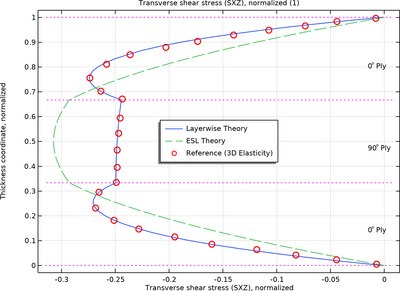# Application Gallery

## Bending of a Simply Supported Composite Laminate

Application ID: 67641

This example demonstrates the bending analysis of a simply supported composite laminate under sinusoidal distributed transverse load. The composite laminate is thick, with a thickness to side length ratio of 1/4. The laminate has three layers in a cross-ply layup. The model is solved using both the Layerwise (LW) theory and the Equivalent Single Layer (ESL) theory.

This is a verification example where the results computed from both theories are compared to the exact 3D elasticity results as given in the reference. The through-thickness variation of the in-plane normal stress, the transverse normal stress, and the transverse shear stresses in each layer is compared to the reference results.This model example illustrates applications of this type that would nominally be built using the following products: